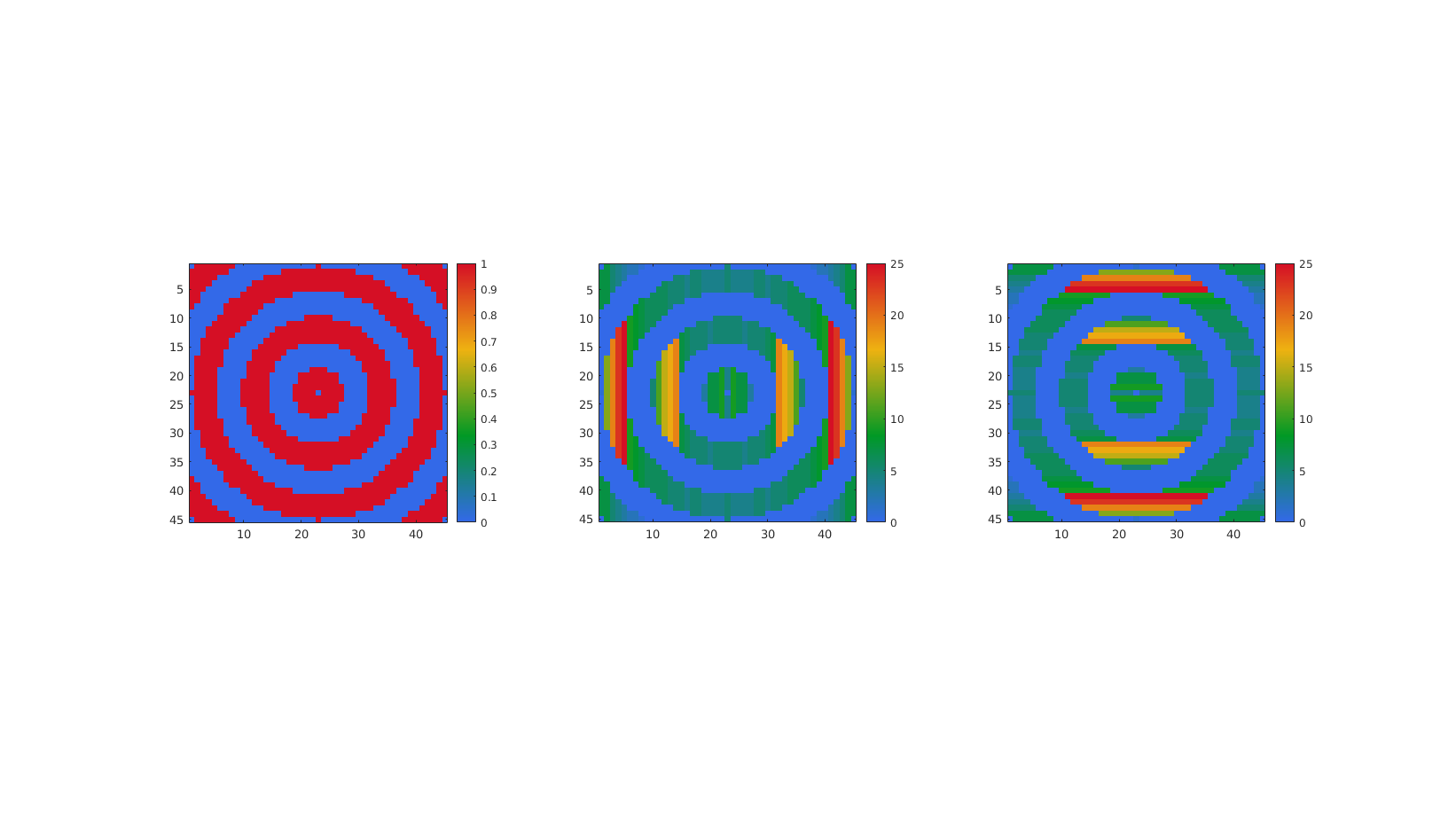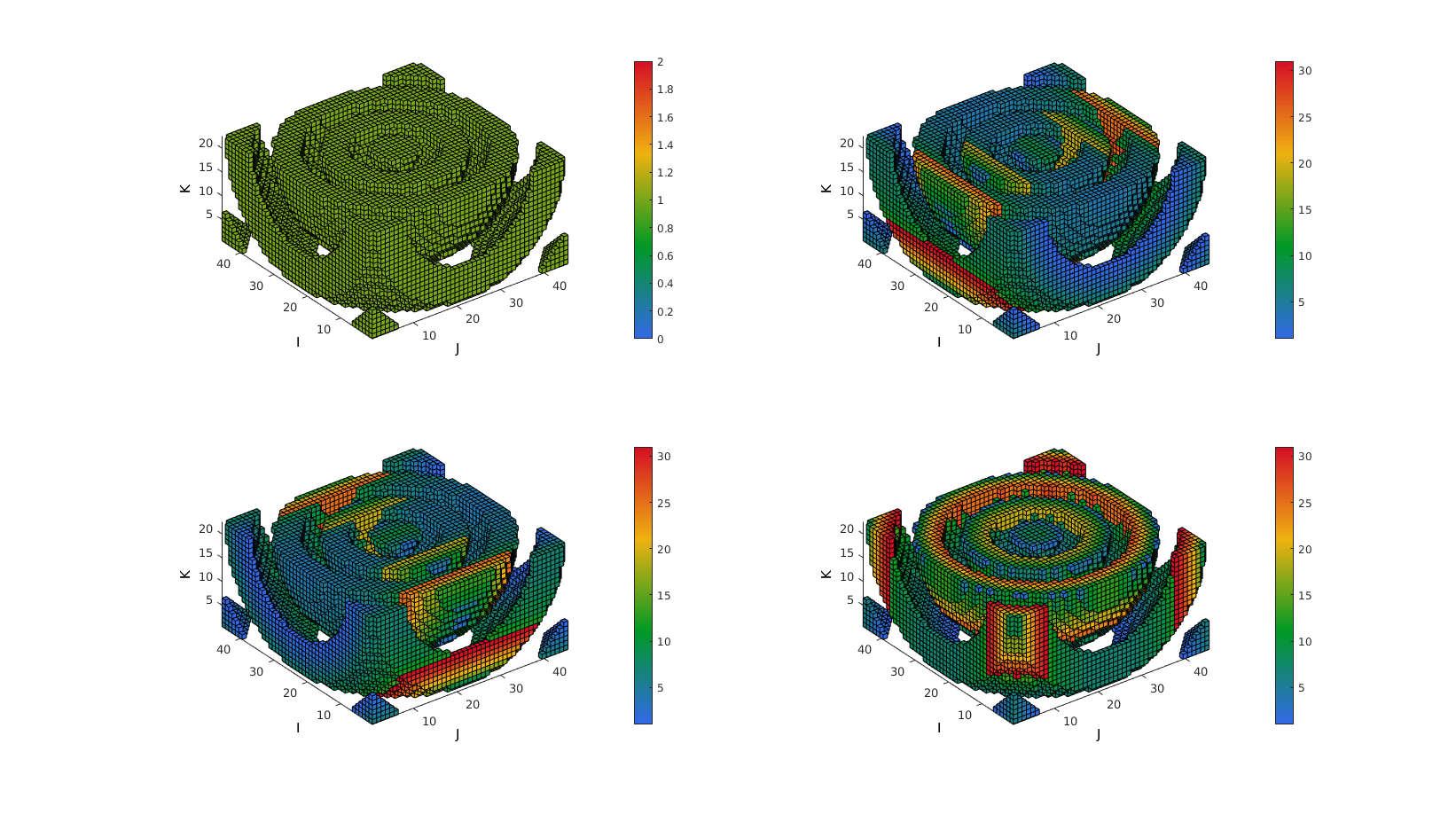Below is a demonstration of the features of the adjacentdircount function

## Description

The adjacentdircount function calculates the number of adjacent entries of an input logic array allong a specified direction.

## Examples

```clear; close all; clc;
```

Plot settings

```fontSize=10;
```

## Example: Using adjacentdircount to compute "connectivity"

The adjacentdircount function computes the number of adjacent elements in the input array that have the value 1. As such it computes a type of connectivity or "thickness" of structures in the logic arrays allong a certain dimension.

Lets consider the following array:

```L=[0 1 1 0 0 1;...
0 0 1 0 0 1;...
1 0 0 1 1 1]; %A logic array or an array containing ones
```

Now view the output of the adjacentdircount function for n=1:

```n=1; %Row direction (i.e. thickness orthogonal to rows)
Lc
```
```Lc =

0     1     2     0     0     3
0     0     2     0     0     3
1     0     0     1     1     3

```

and similarly for for n=2:

```n=2; %Column direction (i.e. thickness orthogonal to columns)
Lc
```
```Lc =

0     2     2     0     0     1
0     0     1     0     0     1
1     0     0     3     3     3

```

## Example: Use for 3D arrays

Create a 3D example array, here L is copied upside down into a second "slice" and again for the third slice.

```L(:,:,2)=flipud(L);
L(:,:,3)=flipud(L(:,:,1));
```
```n=3; %Slice direction (i.e. thickness orthogonal to slices)
Lc
```
```Lc(:,:,1) =

0     1     1     0     0     3
0     0     3     0     0     3
1     0     0     1     1     3

Lc(:,:,2) =

2     0     0     2     2     3
0     0     3     0     0     3
0     2     2     0     0     3

Lc(:,:,3) =

2     0     0     2     2     3
0     0     3     0     0     3
0     2     2     0     0     3

```

## Example: Studying object line feature thickness

```% Simulating a striped image in 3D
np=45;
[X,Y,Z]=meshgrid(linspace(-5*pi,5*pi,np));
M=sin(R);
```

First consider the mid-slice for 2D analysis

```midInd=round(size(M,3)/2);
m=M(:,:,midInd);
```

Convert to a logic array for thickness analysis

```L=m>0;
```

Now use adjacentdircount to compute "connectivity" allong first and second dimensions

```[Lc1]=adjacentdircount(L,1); %1st dim
```

Plotting the results

```hf=cFigure;
hold on;
subplot(1,3,1);
imagesc(L);
colormap gjet; colorbar;
axis equal; axis tight;
set(gca,'FontSize',fontSize);

subplot(1,3,2);
imagesc(Lc1);
colormap gjet; colorbar;
axis equal; axis tight;
set(gca,'FontSize',fontSize);

subplot(1,3,3);
imagesc(Lc2);
colormap gjet; colorbar;
axis equal; axis tight;
set(gca,'FontSize',fontSize);

drawnow;
```Now lets expand to 3D analysis

```L=M>0;

%Construct logic array setting voxels to display using |ind2patch| function
logicPatch=L; %Initialise as L
logicPatch(:,:,midInd:end)=0; %Crop off half to visualize interior

[F,V,C]=ind2patch(logicPatch,L,'vb');
[F1,V1,C1]=ind2patch(logicPatch,Lc1,'vb');
[F2,V2,C2]=ind2patch(logicPatch,Lc2,'vb');
[F3,V3,C3]=ind2patch(logicPatch,Lc3,'vb');

cFigure;
subplot(2,2,1);
xlabel('J');ylabel('I'); zlabel('K'); hold on;
patch('Faces',F,'Vertices',V,'FaceColor','flat','CData',C,'EdgeColor','k');
axis equal; view(3); axis tight; axis vis3d; grid off;
colormap gjet; colorbar;
set(gca,'FontSize',fontSize);

subplot(2,2,2);
xlabel('J');ylabel('I'); zlabel('K'); hold on;
patch('Faces',F1,'Vertices',V1,'FaceColor','flat','CData',C1,'EdgeColor','k');
axis equal; view(3); axis tight; axis vis3d; grid off;
colormap gjet; colorbar;
set(gca,'FontSize',fontSize);

subplot(2,2,3);
xlabel('J');ylabel('I'); zlabel('K'); hold on;
patch('Faces',F2,'Vertices',V2,'FaceColor','flat','CData',C2,'EdgeColor','k');
axis equal; view(3); axis tight; axis vis3d; grid off;
colormap gjet; colorbar;
set(gca,'FontSize',fontSize);

subplot(2,2,4);
xlabel('J');ylabel('I'); zlabel('K'); hold on;
patch('Faces',F3,'Vertices',V3,'FaceColor','flat','CData',C3,'EdgeColor','k');
axis equal; view(3); axis tight; axis vis3d; grid off;
colormap gjet; colorbar;
set(gca,'FontSize',fontSize);

drawnow;
```GIBBON www.gibboncode.org

Kevin Mattheus Moerman, [email protected]

GIBBON footer text

GIBBON: The Geometry and Image-based Bioengineering add-On. A toolbox for image segmentation, image-based modeling, meshing, and finite element analysis.

Copyright (C) 2019 Kevin Mattheus Moerman

This program is free software: you can redistribute it and/or modify it under the terms of the GNU General Public License as published by the Free Software Foundation, either version 3 of the License, or (at your option) any later version.

This program is distributed in the hope that it will be useful, but WITHOUT ANY WARRANTY; without even the implied warranty of MERCHANTABILITY or FITNESS FOR A PARTICULAR PURPOSE. See the GNU General Public License for more details.

You should have received a copy of the GNU General Public License along with this program. If not, see http://www.gnu.org/licenses/.